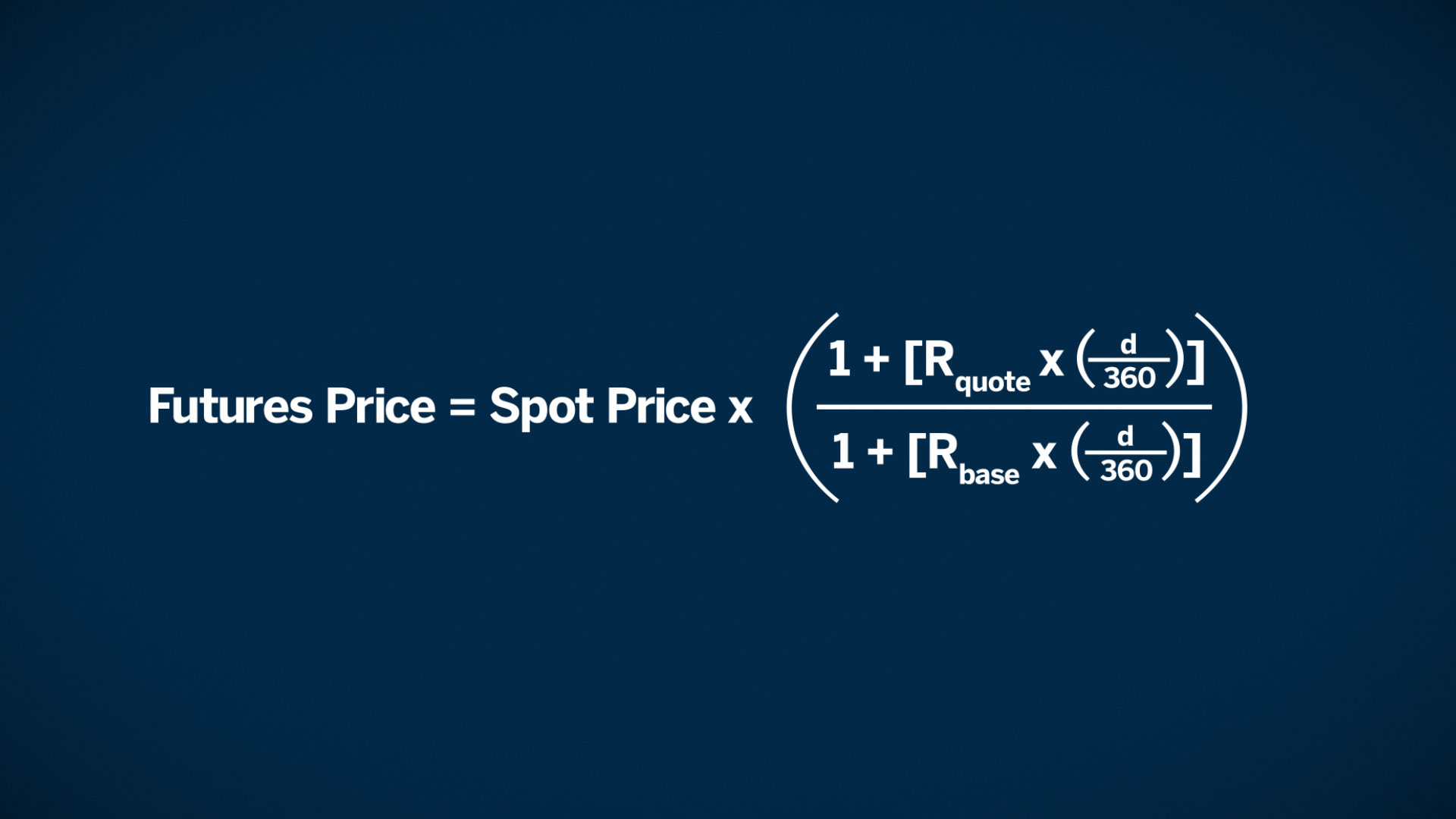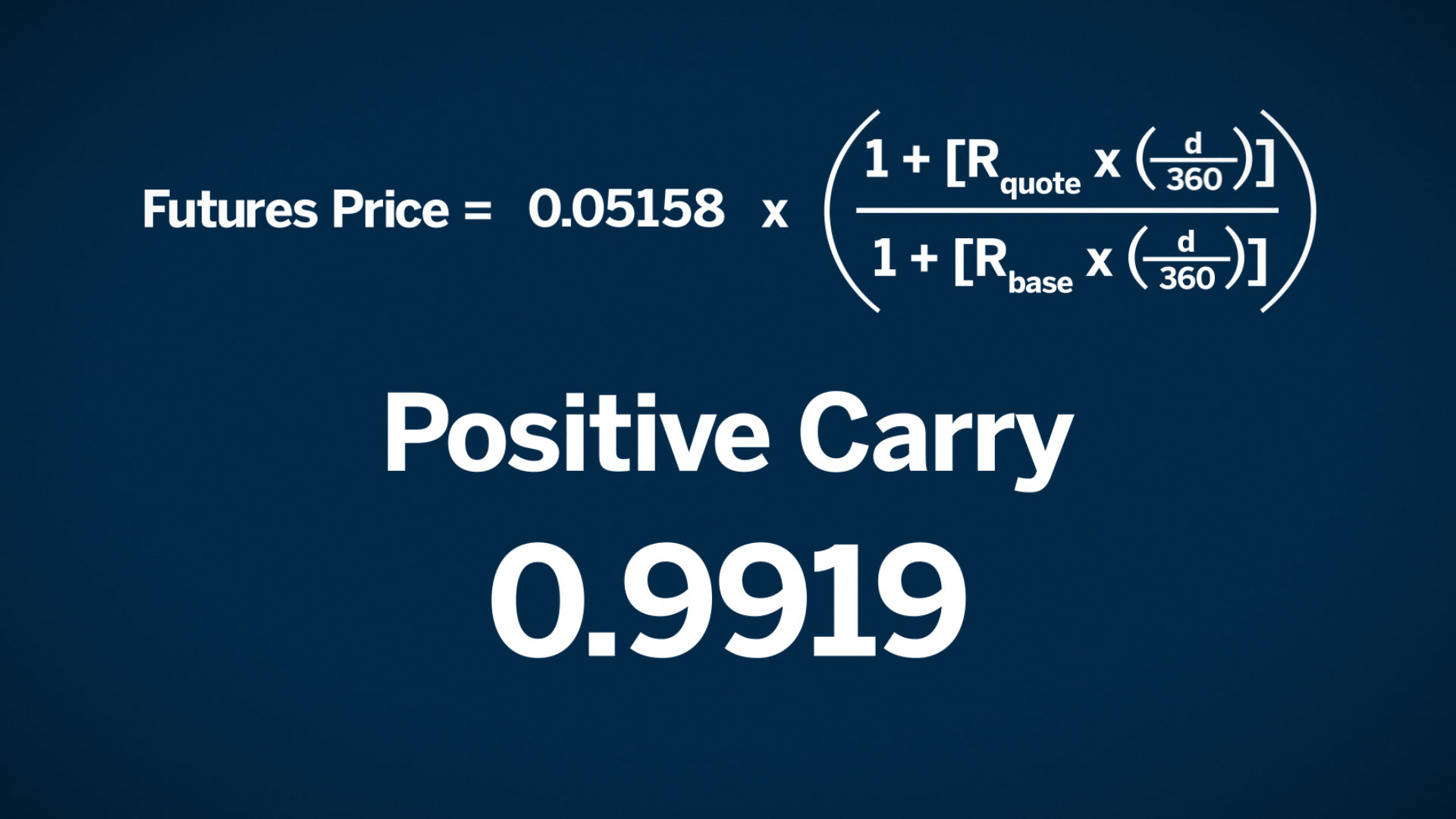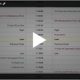# The Importance of FX Futures Pricing and Basis

Understanding FX Basis

The difference between the futures price and spot price of a currency pair is referred to as the basis.

Basis can be either positive or negative. It will depend on the current relationship between the short-term interest rates of the base and terms currencies being considered.

The base currency is the first currency in a currency pair quotation, and the terms currency is the second half of the quotation.

FX Futures Pricing

The price of an FX futures product is based on the currency pair’s spot rate and a short-term interest differential. The pricing formula is similar to how FX forwards are priced in the OTC market. In the following equation, R is the short-term interest rate of a currency and d is the number of days from trade settlement until expiration.If the short-term interest rate of the terms currency rate is lower than the short-term interest rate of the base currency, futures should trade at a discount to the spot price of the currency pair. The basis (that is, futures minus spot) would be quoted as a negative number.

This negative number is the result of what is known as positive carry. It is referred to as positive because an investment in the base currency’s short-term interest rate generates more income than the income generated by the terms currency’s short-term interest rate.Example

Consider Mexican peso/U.S. dollar futures, quoted in American terms as MXN/USD. The Mexican peso resides in the base currency position. Assume it has a short-term interest rate of roughly 4.25% for a term settlement to expiration of 82 days.The USD resides in the terms position and has an equivalent-dated short-term interest rate of 0.70%.

Notice that the USD rate is lower than the Mexican rate. This implies positive carry, so the futures price should be lower than the spot. Using the inputs provided, along with a spot equivalent rate of 0.05158, results in an implied futures price of 0.05116 versus a quoted mid-market price of 0.05115. Futures are quoted at -43 points under spot due to positive carry.Shorter time

Going one step further, we will change the time value to expiration from 82 days to 30 days. How does this effect the multiplier?

Using the same calculation, the new multiplier is 0.99705 which is higher than our multiplier at 82 days. This means the futures price, while still lower, will be closer to spot. This is because of the erosion of the time value of money, or time decay.

As a futures contract approaches expiration, the time value of money runs out and futures price converges toward spot.

The 30-day implied futures price comes to 0.05143 versus a spot of 0.05158. When we subtract the futures price from the spot we get a -15 points.The basis has narrowed from -43 to -15. At expiration, futures and spot will converge to the same level.

Summary

For FX futures, basis is the difference between the futures price and spot price of a currency pairing.

There is a cost of carry consideration for FX futures products. This is a determining factor in whether the futures price trades at a discount or a premium to spot.

If the terms rate is greater than the base rate, futures should trade at a premium to the spot price of the currency. However, when the terms rate is less than the base rate, futures should trade at a discount to spot.

The basis, or difference in futures price versus spot, can be either positive or negative. What is important to remember, is that regardless of whether the basis is positive or negative, the futures price will converge to spot as it approaches expiration. In other words, as time runs out on the futures contract, the interest rate differential has less effect on the futures price and that futures price converges to the spot value.

If you have questions send us a message or schedule an online review .

Regards,

—————————————————————-

Privacy Notice

Disclosure